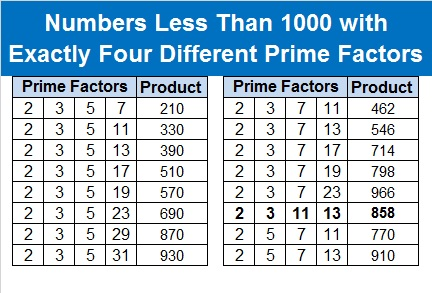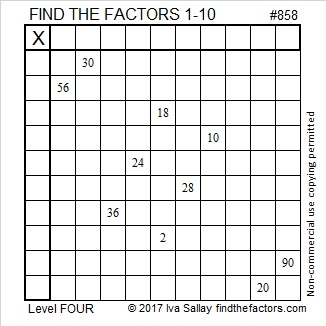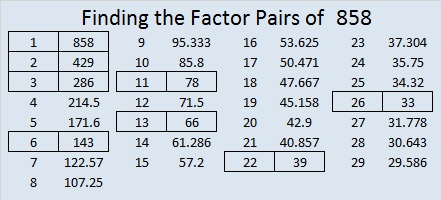# 858 and Level 4

There are sixteen numbers less than 1000 that have four different prime factors. 858 is one of them, and it is the ONLY one that is also a palindrome. Thank you, OEIS.org for alerting us to that fact. No smaller palindrome has four different prime factors!The sixteen products on that chart each have exactly sixteen factors!

Here’s a Find the Factors 1-10 puzzle for you to solve:Print the puzzles or type the solution on this excel file: 10-factors-853-863

Here’s a little more about the number 858:

858 is the hypotenuse of a Pythagorean triple: 330-792-858

• 858 is a composite number.
• Prime factorization: 858 = 2 × 3 × 11 × 13
• The exponents in the prime factorization are 1, 1, 1, and 1. Adding one to each and multiplying we get (1 + 1)(1 + 1)(1 + 1)(1 + 1) = 2 × 2 × 2 × 2 = 16. Therefore 858 has exactly 16 factors.
• Factors of 858: 1, 2, 3, 6, 11, 13, 22, 26, 33, 39, 66, 78, 143, 286, 429, 858
• Factor pairs: 858 = 1 × 858, 2 × 429, 3 × 286, 6 × 143, 11 × 78, 13 × 66, 22 × 39, or 26 × 33
• 858 has no square factors that allow its square root to be simplified. √858 ≈ 29.291637This site uses Akismet to reduce spam. Learn how your comment data is processed.